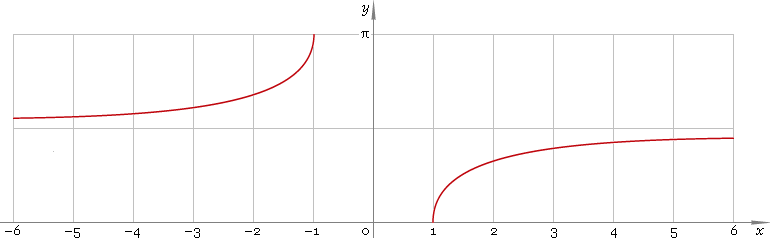The Art of Interface

# arcsec — trigonometric arc secant function

Category. Mathematics.

Abstract. Trigonometric arc secant: definition, plot, properties, identities and table of values for some arguments.

## 1. Definition

Arc secant is inverse of the secant function.

## 2. Plot

Arc secant is discontinuous function defined on entire real axis except the (−1, 1) range — so, its domain is (−∞, −1]∪[1, +∞). Function plot is depicted below — fig. 1.Fig. 1. Plot of the arc secant function y = arcsecx.

Function codomain is limited to the range [0, π/2)∪(π/2, π].

## 3. Identities

Complementary angle:

arcsecx + arccscx = π/2

and as consequence:

arcsec csc φ = π/2 − φ

Negative argument:

arcsec(−x) = π − arcsecx

Reciprocal argument:

arsec(1/x) = arccosx

Sum and difference:

arcsecx + arcsecy = arcsec(xy /{1 − xy√[(1 − 1 /x2)(1 − 1 /y2)]})
arcsecx − arcsecy = arcsec(xy /{1 + xy√[(1 − 1 /x2)(1 − 1 /y2)]})

Some argument values:

Argument xValue arcsecx
10
√6 − √2π/12
√(50 − 10√5) /5π/10
√(2 − √2)π/8
2√3 /3π/6
√5 − 1π/5
√2π/4
√(50 + 10√5) /53π/10
2π/3
√(4 + 2√2)3π/8
√5 + 12π/5
√6 + √25π/12
Table 1. Arc secant for some argument values.

## 4. Support

Trigonometric arc secant function arcsec of the real argument is supported by free version of the Librow calculator.

Trigonometric arc secant function arcsec of the complex argument is supported by professional version of the Librow calculator.

## 5. How to use

To calculate arc secant of the number:

``arcsec(-1);``

To calculate arc secant of the current result:

``arcsec(rslt);``

To calculate arc secant of the number x in memory:

``arcsec(mem[x]);``